• No products in the cart.

# MTH4: CONSTRUCTION AND LOCI PLUS VARIATIONS

## CONSTRUCTION AND LOCI

A locus of a point is the path it takes as it moves following the given conditions. (Law)

## Loci

This section covers Loci within Geometry and Measures.

A locus is a set of points satisfying a certain condition. For example, the locus of points that are 1cm from the origin is a circle of radius 1cm centred on the origin, since all points on this circle are 1cm from the origin.

N.B. if a point P is ‘equidistant’ from two points A and B, then the distance between P and A is the same as the distance between P and B, as illustrated here: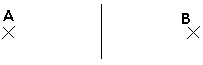The points on the line are equidistant from A and B

Don’t let the term ‘locus’ put you off. Questions on loci (which is the plural of locus) often don’t use the term.

Example

The diagram shows two points P and Q. On the diagram shade the region which contains all the points which satisfy both the following: the distance from P is less than 3cm, the distance from P is greater than the distance from Q.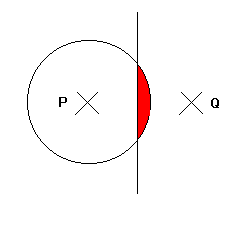All of the points on the circumference of the circle are 3cm from P. Therefore all of the points satisfying the condition that the distance from P is less than 3cm are in the circle.
If we draw a line in the middle of P and Q, all of the points on this line will be the same distance from P as they are from Q. They will be therefore closer to Q, and further away from P, if they are on the right of such a line.

Therefore all of the points satisfying both of these conditions are shaded in red.

Three important loci

The word locus describes the position of points which obey a certain rule.

Three important loci are:

• The circle – the locus of points which are equidistant from a fixed point, the centre.
• The perpendicular bisector – the locus of points which are equidistant from two fixed points A and B.
• The angle bisector – the locus of points which are equidistant from two fixed lines.

Example:

The diagram shows the walls of a rectangular shed, ABCD, measuring 8m by 5m. A goat is tied to the corner of C by a rope 6m long.

The shaded area shows the part the goat can reach.

Examples.

1. Draw a line XY of a length 10 cm. Construct the locus of a point which is equidistant from x and y.
2. Draw two lines AB and AC of length 8 cm, where , BAC = 600. Construct the locus of appoint which is equidistant from the lines AB and AC.
3. Draw a circle , centre O of radius 5 cm and a radius OA. Construct the locus of appoint P. which moves so that OAP = 900
4. Draw a line AB of length 10 cm and construct the circle with diameter AB. Indicate the locus of a point P which moves to that APB = 900
Exercise
1. Inspector Clouseau has put a radio transmitter on a suspects car, which is parked some where In Gayaza. From the strength of the signals received at Points R and P, Clouseau knows that the car is
a) not more than 10 km from R and
b) not more than 20 km from R
Make a scale drawing (1 cm=10 km) and show the possible positions of the car .

A VIDEO BELOW ABOUT CONSTRUCTION AND LOCI

VARIATIONS

Mathematical equations display some type of parameters when it established a relationship. There are normally two types of quantities exist. One is constant. It doesn’t change with the changes in other parameters in the equation.However, others are the variables which change in different situations. The changing of variable parameters is often gone by the term variations or algebraic variations.

Let’s explain with an example of a simple equation y = mx wherein m refers to a constant. Further, if we assume that the value of m is 5, then, in this case, the equation becomes y = 5x.

Similarly, when x = 1, y = 1 × 5 = 5

Further, when x = 2, y = 2 × 5 = 10

And when x = 3, y = 3 × 5 = 15

By simply changing the value of y, x gets different values as well. This is the variation of y with different values of x and similarly you can show that with different values of y the value of x changes.

Variations can be of different types according to the pattern of changing or relationships of variables.

Proportions

# Proportions

Proportion says that two ratios (or fractions) are equal.

### Example:So 1-out-of-3 is equal to 2-out-of-6

The ratios are the same, so they are in proportion.

### Example: Rope

A rope’s length and weight are in proportion.

When 20m of rope weighs 1kg, then:

• 40m of that rope weighs 2kg
• 200m of that rope weighs 10kg
• etc.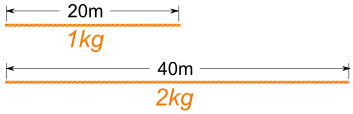So:

201 = 402

### Sizes

When shapes are “in proportion” their relative sizes are the same.

 Here we see that the ratios of head length to body length are the same in both drawings.So they are proportional.Making the head too long or short would look bad!### Example: International paper sizes (like A3, A4, A5, etc) all have the same proportions:So any artwork or document can be resized to fit on any sheet. Very neat.

## Working With Proportions

NOW, how do we use this?

### Example: you want to draw the dog’s head … how long should it be?Let us write the proportion with the help of the 10/20 ratio from above:

?42 = 1020

Now we solve it using a special method:Multiply across the known corners,
then divide by the third number

And we get this:

? = (42 × 10) / 20
= 420 / 20
21

So you should draw the head 21 long.

## Using Proportions to Solve Percents

A percent is actually a ratio! Saying “25%” is actually saying “25 per 100”:

25% = 25100

We can use proportions to solve questions involving percents.

The trick is to put what we know into this form:

PartWhole = Percent100

### Example: what is 25% of 160 ?

The percent is 25, the whole is 160, and we want to find the “part”:

Part160 = 25100

Multiply across the known corners, then divide by the third number:Part = (160 × 25) / 100
= 4000 / 100
40

Answer: 25% of 160 is 40.

Note: we could have also solved this by doing the divide first, like this:

Part = 160 × (25 / 100)
= 160 × 0.25
40

Either method works fine.

We can also find a Percent:

### Example: what is \$12 as a percent of \$80 ?

Fill in what we know:

\$12\$80 = Percent100

Multiply across the known corners, then divide by the third number. This time the known corners are top left and bottom right:Percent = (\$12 × 100) / \$80
= 1200 / 80
15%

Answer: \$12 is 15% of \$80

Or find the Whole:

### Example: The sale price of a phone was \$150, which was only 80% of normal price. What was the normal price?

Fill in what we know:

\$150Whole = 80100

Multiply across the known corners, then divide by the third number:Whole = (\$150 × 100) / 80
= 15000 / 80
187.50

Answer: the phone’s normal price was \$187.50

## Using Proportions to Solve Triangles

We can use proportions to solve similar triangles.

### Example: How tall is the Tree?

Sam tried using a ladder, tape measure, ropes and various other things, but still couldn’t work out how tall the tree was.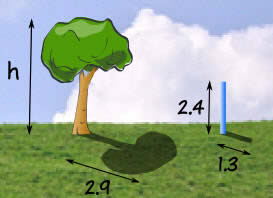But then Sam has a clever idea … similar triangles!

Sam measures a stick and its shadow (in meters), and also the shadow of the tree, and this is what he gets: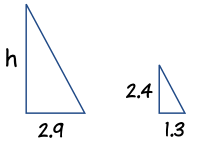Now Sam makes a sketch of the triangles, and writes down the “Height to Length” ratio for both triangles:

Height:Shadow Length:     h2.9 m = 2.4 m1.3 m

Multiply across the known corners, then divide by the third number:

h = (2.9 × 2.4) / 1.3
= 6.96 / 1.3
5.4 m (to nearest 0.1)

Answer: the tree is 5.4 m tall.

And he didn’t even need a ladder!

The “Height” could have been at the bottom, so long as it was on the bottom for BOTH ratios, like this:Let us try the ratio of “Shadow Length to Height”:

Shadow Length:Height:     2.9 mh = 1.3 m2.4 m

Multiply across the known corners, then divide by the third number:

h = (2.9 × 2.4) / 1.3
= 6.96 / 1.3
5.4 m (to nearest 0.1)

It is the same calculation as before.

## A “Concrete” Example

Ratios can have more than two numbers!

For example concrete is made by mixing cement, sand, stones and water.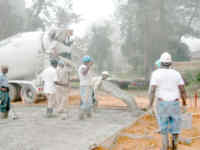A typical mix of cement, sand and stones is written as a ratio, such as 1:2:6.

We can multiply all values by the same amount and still have the same ratio.

10:20:60 is the same as 1:2:6

So when we use 10 buckets of cement, we should use 20 of sand and 60 of stones.

### Example: you have just put 12 buckets of stones into a mixer, how much cement and how much sand should you add to make a 1:2:6 mix?

Let us lay it out in a table to make it clearer:

CementSandStones
Ratio Needed:126
You Have:12

You have 12 buckets of stones but the ratio says 6.

That is OK, you simply have twice as many stones as the number in the ratio … so you need twice as much of everything to keep the ratio.

Here is the solution:

CementSandStones
Ratio Needed:126
You Have:2412

And the ratio 2:4:12 is the same as 1:2:6 (because they show the same relative sizes)

So the answer is: add 2 buckets of Cement and 4 buckets of Sand. (You will also need water and a lot of stirring….)

Why are they the same ratio? Well, the 1:2:6 ratio says to have:

• twice as much Sand as Cement (1:2:6)
• 6 times as much Stones as Cement (1:2:6)

In our mix we have:

• twice as much Sand as Cement (2:4:12)
• 6 times as much Stones as Cement (2:4:12)

So it should be just right!

That is the good thing about ratios. You can make the amounts bigger or smaller and so long as the relative sizes are the same then the ratio is the same.

A VIDEO BELOW ABOUT DIRECT AND INVERSE VARIATION

## Part Variation

If two variables x and y are related by a formula such as y = a + bx, then y is said to vary partly as the constant a and partly as x.For example, if a taxi is hired, then the cost of travelling from one place to another may be considered as the sum of two quantities, of which one is constant and the other varies directly as the time taken to reach the destination. That is: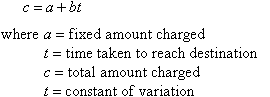###### Note:

part variation keeps a direct variation but includes an extra fixed constant.

Graphically, this relation takes the shape of a straight line with gradient equal to the value of and the vertical intercept equal to the value of a.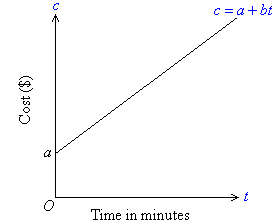#### Example 13

The cost, c, of publishing a bulletin consists of two parts:

• a fixed charge, a, of \$15; and
• a charge for publishing and the paper, proportional to the number of copies published, n.

If the cost of 500 copies is \$35, find the cost of 1000 copies.

##### Solution: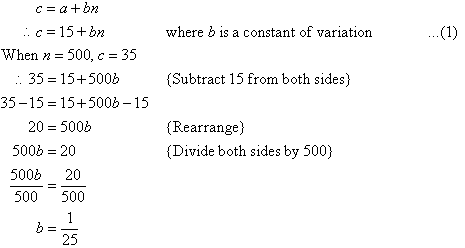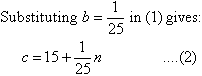This is the required formula.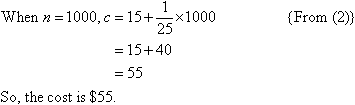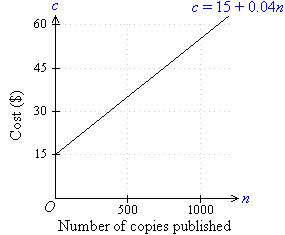#### Attachments60

• Missing Attachment
• Missing Attachment
• Missing Attachment
• Missing Attachment
• Missing Attachment
• Missing Attachment
• Missing Attachment
• Missing Attachment
• Missing Attachment
• Missing Attachment
• Missing Attachment
• Missing Attachment
• Missing Attachment
• Missing Attachment
• Missing Attachment
• Missing Attachment
• Missing Attachment
• Missing Attachment
• Missing Attachment
• Missing Attachment
• Missing Attachment
• Missing Attachment
• Missing Attachment
• Missing Attachment
• Missing Attachment
• Missing Attachment
• Missing Attachment
• Missing Attachment
• Missing Attachment
• Missing Attachment
• Missing Attachment
• Missing Attachment
• Missing Attachment
• Missing Attachment
• Missing Attachment
• Missing Attachment
• Missing Attachment
• Missing Attachment
• Missing Attachment
• Missing Attachment
• Missing Attachment
• Missing Attachment
• Missing Attachment
• Missing Attachment
• Missing Attachment
• Missing Attachment
• Missing Attachment
• Missing Attachment
• Missing Attachment
• Missing Attachment
• Missing Attachment
• Missing Attachment
• Missing Attachment
• Missing Attachment
• Missing Attachment
• Missing Attachment
• Missing Attachment
• Missing Attachment
• Missing Attachment
• Missing Attachment

### ASSIGNMENT : CONSTRUCTION AND LOCI PLUS VARIATIONS ASSIGNMENTMARKS : 50  DURATION : 3 hours

Yaaka DN © Ultimate Multimedia Consult